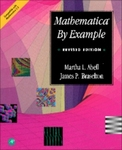## Mathematical Sciences Faculty Bookshelf#### Title

Mathematica By Example

#### Files

Download Full Text

#### Description

Georgia Southern faculty members Martha L. Abell and James P. Braselton co-authored Mathematica by Example, Revised Edition.

Mathematica by Example, Revised Edition presents the commands and applications of Mathematica, a system for doing mathematics on a computer. This text serves as a guide to beginning users of Mathematica and users who do not intend to take advantage of the more specialized applications of Mathematica.

The book combines symbolic manipulation, numerical mathematics, outstanding graphics, and a sophisticated programming language. It is comprised of 7 chapters. Chapter 1 gives a brief background of the software and how to install it in the computer. Chapter 2 introduces the essential commands of Mathematica. Basic operations on numbers, expressions, and functions are introduced and discussed. Chapter 3 provides Mathematica's built-in calculus commands. The fourth chapter presents elementary operations on lists and tables. This chapter is a prerequisite for Chapter 5 which discusses nested lists and tables in detail. The purpose of Chapter 6 is to illustrate various computations Mathematica can perform when solving differential equations. Chapter 7 discusses some of the more frequently used commands contained in various graphics packages available with Mathematica.

Engineers, computer scientists, physical scientists, mathematicians, business professionals, and students will find the book useful.

2-17-1994

Academic Press

#### ISBN for this edition (13-digit)

9781483213903COinS